#### The Bug1 strategy

A strategy called Bug1 was developed in  and is illustrated in Figure 12.20. The execution is as follows:

1. Move toward the goal until an obstacle or the goal is encountered. If the goal is reached, then stop.
2. Turn left and follow the entire perimeter of the contacted obstacle. Once the full perimeter has been visited, then return to the point at which the goal was closest, and go to Step 1.
Determining that the entire perimeter has been traversed may seem to require a pebble or marker; however, this can be inferred by finding the point at which the goal sensor reading repeats.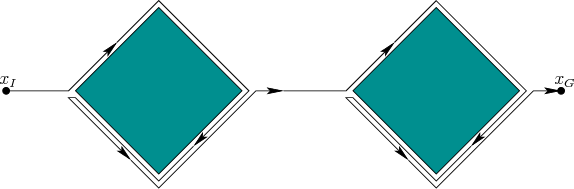The worst case is conceptually simple to understand. The total distance traveled by the robot is no greater than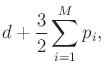(12.25)

in which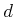is the Euclidean distance from the initial position to the goal position,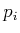is the perimeter of theth obstacle, andis the number of obstacles. This means that the boundary of each obstacle is followed no more than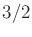times. Figure 12.21 shows an example in which each obstacle is traversedtimes. This bound relies on the fact that the robot can always recall the shortest path along the boundary to the point from which it needs to leave. This seems reasonable because the robot can infer its distance traveled along the boundary from the goal sensor. If this was not possible, then thewould have to be replaced by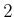because the robot could nearly traverse the full boundary twice in the worst case.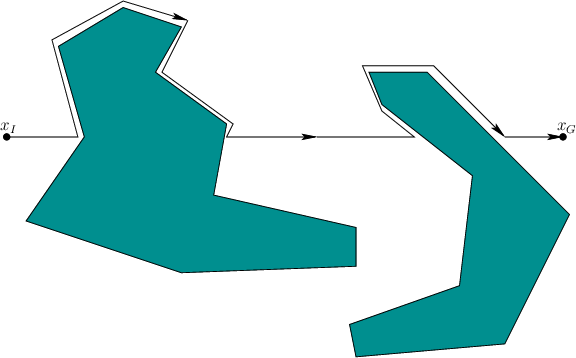Steven M LaValle 2020-08-14